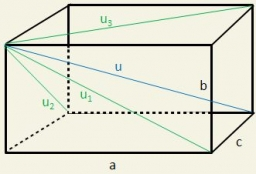# Rectangle 7768

The base of a cuboid is a rectangle. The ratio of its length to width is 3:2. The length of the rectangle of the base is in the ratio of 4:5 to the height of the block. The sum of the lengths of all the edges of the block is 2.8m. Find:
a) the surface of the cuboid in cm2
b) volume in dm3

S =  50688 cm2
V =  737280 cm3

### Step-by-step explanation:Did you find an error or inaccuracy? Feel free to write us. Thank you!

Tips for related online calculators
Check out our ratio calculator.
Do you have a linear equation or system of equations and looking for its solution? Or do you have a quadratic equation?
Do you want to convert length units?
Do you know the volume and unit volume, and want to convert volume units?

#### Grade of the word problem:

We encourage you to watch this tutorial video on this math problem: